SNAP Library 6.0, Developer Reference  2020-12-09 16:24:20 SNAP, a general purpose, high performance system for analysis and manipulation of large networks
TLocClust Class Reference

Local Spectral Clustering algorithm. More...

`#include <ncp.h>`

Collaboration diagram for TLocClust: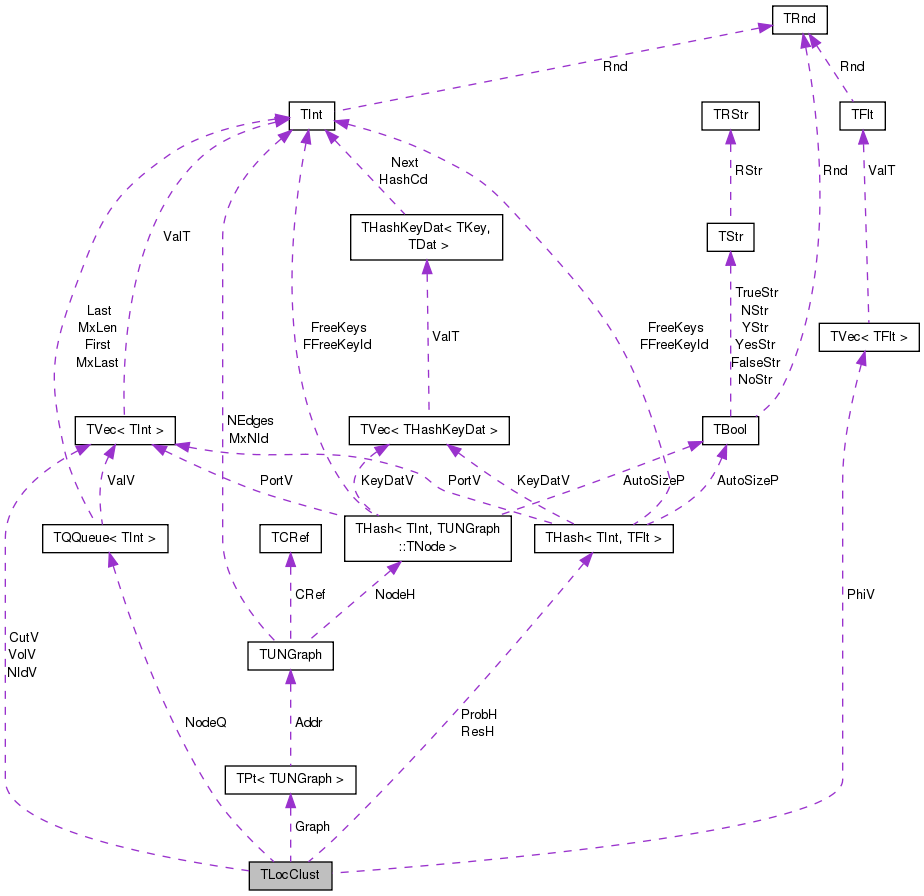[legend]

## Public Member Functions

TLocClust (const PUNGraph &GraphPt, const double &AlphaVal)

int Len () const
Returns the support of the approximate random walk, the number of nodes with non-zero PageRank score. More...

int GetRndWalkSup () const
Returns the support of the approximate random walk, the number of nodes with non-zero PageRank score. More...

int GetNId (const int &NodeN) const
Returns the ID of the NodeN-th node in the sweep vector. More...

int GetVol (const int &Nodes) const
Returns the volume of the set of first NodeN nodes in the sweep vector. More...

int GetCut (const int &Nodes) const
Returns the size of the cut of the first Nodes nodes in the sweep vector. More...

double GetPhi (const int &ValId) const
Returns the conductance of the cut separating the first Nodes nodes in the sweep vector from the rest of the graph. More...

const TIntVGetNIdV () const
Vector of node IDs sorted in the random walk order. More...

const TIntVGetVolV () const
Volume (sum of the degrees) of first K-nodes in the node-id vector (GetNIdV()). More...

const TIntVGetCutV () const
Edges cut to separate the first K-nodes in the node-id vector (GetNIdV()) from the rest of the graph. More...

const TFltVGetPhiV () const
Conducatance of the cut separating first K-nodes in the node-id vector (GetNIdV()) from the rest of the graph. More...

int BestCut () const
Index K of the cut of the minimum conductance around the seed node. More...

int BestCutNodes () const
Number of nodes inside the 'best' (minimum conductance) cut. More...

int GetCutEdges () const
Number of edges in the 'best' (minimum conductance) cut. More...

int GetCutVol () const
Volume of the 'best' (minimum conductance) cut. More...

double GetCutPhi () const
Conductance of the 'best' (minimum conductance) cut. More...

int ApproxPageRank (const int &SeedNode, const double &Eps)
Computes Approximate PageRank from the seed node SeedNId and with tolerance Eps. More...

void SupportSweep ()
After the function ApproxPageRank() has been run the SupportSweep() computes the volume, cut size, node ids, conductance vectors. More...

void FindBestCut (const int &SeedNode, const int &ClustSz, const double &MinSizeFrac=0.2)
Finds minimum conductance cut in the graph around the seed node. More...

void PlotVolDistr (const TStr &OutFNm, TStr Desc=TStr()) const
Plots the cluster volume vs. cluster size K (cluster is composed of nodes NIdV[1...K]). More...

void PlotCutDistr (const TStr &OutFNm, TStr Desc=TStr()) const
Plots the cluster cut size vs. cluster size K (cluster is composed of nodes NIdV[1...K]). More...

void PlotPhiDistr (const TStr &OutFNm, TStr Desc=TStr()) const
Plots the cluster conductance vs. cluster size K (cluster is composed of nodes NIdV[1...K]). More...

void SavePajek (const TStr &OutFNm) const
Saves the network in the Pajek format so it can be visualized. Red node represents the seed and color the cluster membership. More...

## Static Public Member Functions

static void DrawWhiskers (const PUNGraph &Graph, TStr FNmPref, const int &PlotN)
Draws the 'whiskers' of the graph. Whiskers are small sub-graphs that are attached to the rest of the graph via a single edge. More...

static void GetCutStat (const PUNGraph &Graph, const TIntV &NIdV, int &Vol, int &Cut, double &Phi, int GraphEdges=-1)
For a given Graph and a set of nodes NIdV the function returns the Volume, CutSize and the Conductance of the cut. More...

static void GetCutStat (const PUNGraph &Graph, const TIntSet &NIdSet, int &Vol, int &Cut, double &Phi, int GraphEdges=-1)
For a given Graph and a set of nodes NIdV the function returns the Volume, CutSize and the Conductance of the cut. More...

static void PlotNCP (const PUNGraph &Graph, const TStr &FNm, const TStr Desc="", const bool &BagOfWhiskers=true, const bool &RewireNet=false, const int &KMin=10, const int &KMax=Mega(100), const int &Coverage=10, const bool &SaveTxtStat=false, const bool &PlotBoltzman=false)

## Static Public Attributes

static bool Verbose = true

PUNGraph Graph

int Nodes

int Edges2

TIntFltH ProbH

TIntFltH ResH

TIntQ NodeQ

double Alpha

int SeedNId

TIntV NIdV

TIntV VolV

TIntV CutV

TFltV PhiV

int BestCutIdx

## Friends

class TLocClustStat

## Detailed Description

Local Spectral Clustering algorithm.

The code implements the PageRank Nibble local clustering algorithm of Andersen, Chung and Lang. Given a single starting seed node, the algorithm will then find the clusters around that node. This is achieved by the algorithm finding the approximate personalized PageRank score of every node with respect to the Seed node. Nodes are then ordered by the PageRank score and the idea is then that by 'sweeping' the vector of PageRank scores one can find communities around the chosen seed node. The idea is to try out K = 1...N/2 and then for a set of {node_1 ... node_K} test the value of the conductance (Phi). If the conductance at certain value of K achieves a local minima, then we found a good cut in the graph. This method is also used for computing the Network Community Profile plots. See: Local Graph Partitioning using PageRank Vectors by R. Andersen, F. Chung and K. Lang URL: http://www.math.ucsd.edu/~fan/wp/localpartition.pdf

Definition at line 24 of file ncp.h.

## Constructor & Destructor Documentation

 TLocClust::TLocClust ( const PUNGraph & GraphPt, const double & AlphaVal )
inline

Definition at line 39 of file ncp.h.

39  :
40  Graph(GraphPt), Nodes(GraphPt->GetNodes()), Edges2(2*GraphPt->GetEdges()), Alpha(AlphaVal) { }
int Edges2
Definition: ncp.h:29
int Nodes
Definition: ncp.h:29
double Alpha
Definition: ncp.h:32
PUNGraph Graph
Definition: ncp.h:28

## Member Function Documentation

 int TLocClust::ApproxPageRank ( const int & SeedNode, const double & Eps )

Computes Approximate PageRank from the seed node SeedNId and with tolerance Eps.

The algorithm basically sets the PageRank scores of nodes with score <Eps to zero. So the lower the value of Eps the longer the algorithm will run.

Definition at line 9 of file ncp.cpp.

Referenced by FindBestCut().

9  {
10  for (int i = 0; i < ProbH.Len(); i++) { ProbH[i]=0.0; }
11  for (int i = 0; i < ResH.Len(); i++) { ResH[i]=0.0; }
12  ProbH.Clr(false, -1, false);
13  ResH.Clr(false, -1, false);
15  int iter = 0;
16  double OldRes = 0.0;
17  NodeQ.Clr(false);
18  NodeQ.Push(SeedNode);
19  TExeTm ExeTm;
20  while (! NodeQ.Empty()) {
21  const int NId = NodeQ.Top(); NodeQ.Pop();
22  const TUNGraph::TNodeI& Node = Graph->GetNI(NId);
23  const int NIdDeg = Node.GetOutDeg();
24  const double PushVal = ResH.GetDat(NId) - 0.5*Eps*NIdDeg;
25  const double PutVal = (1.0-Alpha) * PushVal / double(NIdDeg);
27  ResH.AddDat(NId) = 0.5 * Eps * NIdDeg;
28  for (int e = 0; e < NIdDeg; e++) {
29  const int DstNId = Node.GetOutNId(e);
30  const int DstDeg = Graph->GetNI(DstNId).GetOutDeg();
32  OldRes = ResVal;
33  ResVal += PutVal;
34  if (ResVal >= Eps*DstDeg && OldRes < Eps*DstDeg) {
35  NodeQ.Push(DstNId); }
36  }
37  iter++;
38  if (iter % Mega(1) == 0) {
39  printf(" %d[%s]", NodeQ.Len(), ExeTm.GetStr());
40  if (iter/1000 > Graph->GetNodes() || ExeTm.GetSecs() > 4*3600) { // more than 2 hours
41  printf("Too many iterations! Stop to save time.\n");
42  return iter; }
43  }
44  }
45  // check that the residuals are sufficiently small
46  /*for (int i =0; i < ProbH.Len(); i++) {
47  const int Deg = Graph->GetNI(ResH.GetKey(i)).GetOutDeg();
48  IAssert(ResH[i] < Eps*Deg); } //*/
49  return iter;
50 }
TIntFltH ResH
Definition: ncp.h:30
Definition: tm.h:355
bool Empty() const
Definition: ds.h:2646
double Val
Definition: dt.h:1388
Node iterator. Only forward iteration (operator++) is supported.
Definition: graph.h:68
const TDat & GetDat(const TKey &Key) const
Definition: hash.h:262
int GetNodes() const
Returns the number of nodes in the graph.
Definition: graph.h:192
void Pop()
Definition: ds.h:2650
int GetOutDeg() const
Returns out-degree of the current node (returns same as value GetDeg() since the graph is undirected)...
Definition: graph.h:94
TNodeI GetNI(const int &NId) const
Returns an iterator referring to the node of ID NId in the graph.
Definition: graph.h:241
#define Mega(n)
Definition: gbase.h:4
int Len() const
Definition: ds.h:2647
const TVal & Top() const
Definition: ds.h:2648
double Alpha
Definition: ncp.h:32
int GetOutNId(const int &NodeN) const
Returns ID of NodeN-th out-node (the node the current node points to).
Definition: graph.h:106
PUNGraph Graph
Definition: ncp.h:28
TIntQ NodeQ
Definition: ncp.h:31
double GetSecs() const
Definition: tm.h:366
void Clr(const bool &DoDel=true, const int &NoDelLim=-1, const bool &ResetDat=true)
Definition: hash.h:361
void Push(const TVal &Val)
Definition: ds.h:2653
void Clr(const bool &DoDel=true)
Definition: ds.h:2635
const char * GetStr() const
Definition: tm.h:368
int Len() const
Definition: hash.h:228
Definition: hash.h:238
TIntFltH ProbH
Definition: ncp.h:30

Here is the call graph for this function: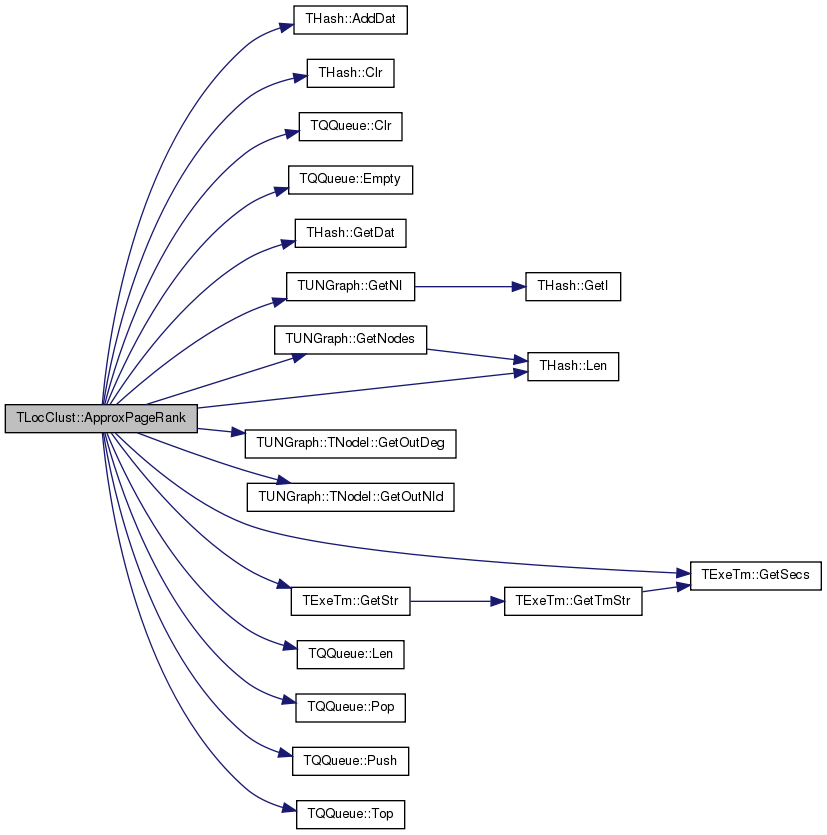Here is the caller graph for this function:int TLocClust::BestCut ( ) const
inline

Index K of the cut of the minimum conductance around the seed node.

This means that the set of GetNId(0)...GetNId(K) forms the best cut around the seed node.

Definition at line 73 of file ncp.h.

References BestCutIdx.

Referenced by GetCutEdges(), GetCutPhi(), GetCutVol(), and SavePajek().

73 { return BestCutIdx; }
int BestCutIdx
Definition: ncp.h:37

Here is the caller graph for this function: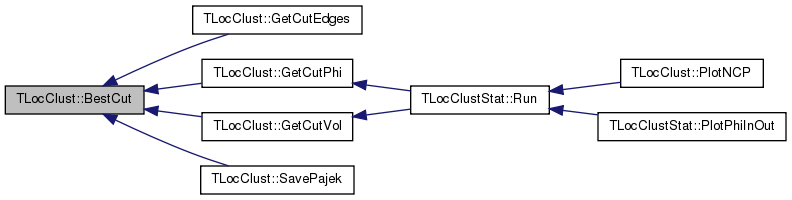int TLocClust::BestCutNodes ( ) const
inline

Number of nodes inside the 'best' (minimum conductance) cut.

Definition at line 75 of file ncp.h.

Referenced by TLocClustStat::Run(), and SavePajek().

75 { return BestCutIdx+1; }
int BestCutIdx
Definition: ncp.h:37

Here is the caller graph for this function:void TLocClust::DrawWhiskers ( const PUNGraph & Graph, TStr FNmPref, const int & PlotN = `10` )
static

Draws the 'whiskers' of the graph. Whiskers are small sub-graphs that are attached to the rest of the graph via a single edge.

Definition at line 172 of file ncp.cpp.

172  {
173  TCnComV CnComV; TSnap::Get1CnCom(Graph, CnComV);
174  CnComV.Sort(false);
175  // plot size distribution
176  { TIntH SzCntH;
177  for (int i = 0; i < CnComV.Len(); i++) { SzCntH.AddDat(CnComV[i].Len()) += 1; }
178  TGnuPlot::PlotValCntH(SzCntH, "whiskSz."+FNmPref, TStr::Fmt("%s. G(%d, %d)", FNmPref.CStr(), Graph->GetNodes(),
179  Graph->GetEdges()), "Whisker size (Maximal component connected with a bridge edge)", "Count", gpsLog10XY, false); }
180  // draw them
181  int BrNodeId = -1;
182  for (int c = 0; c < TMath::Mn(CnComV.Len(), PlotN); c++) {
183  const PUNGraph BrClust = TSnap::GetSubGraph(Graph, CnComV[c].NIdV);
184  for (TUNGraph::TNodeI NI = BrClust->BegNI(); NI < BrClust->EndNI(); NI++) {
185  if (NI.GetOutDeg() != Graph->GetNI(NI.GetId()).GetOutDeg()) { BrNodeId=NI.GetId(); break; } }
187  TSnap::DrawGViz(BrClust, gvlNeato, TStr::Fmt("whisk-%s-%02d.gif", FNmPref.CStr(), c+1),
188  TStr::Fmt("Bridge node id: %d, n=%d, e=%d", BrNodeId, BrClust->GetNodes(), BrClust->GetEdges()), false, ClrH);
189  }
190 }
void DrawGViz(const PGraph &Graph, const TGVizLayout &Layout, const TStr &PltFNm, const TStr &Desc=TStr(), const bool &NodeLabels=false, const TIntStrH &NIdColorH=TIntStrH())
Draws a given Graph using a selected GraphViz Layout engine with nodes colored.
Definition: gviz.h:34
static const T & Mn(const T &LVal, const T &RVal)
Definition: xmath.h:36
TSizeTy Len() const
Returns the number of elements in the vector.
Definition: ds.h:575
Node iterator. Only forward iteration (operator++) is supported.
Definition: graph.h:68
int Len() const
Returns the support of the approximate random walk, the number of nodes with non-zero PageRank score...
Definition: ncp.h:42
Definition: gviz.h:3
void Sort(const bool &Asc=true)
Sorts the elements of the vector.
Definition: ds.h:1318
PUNGraph GetSubGraph(const PUNGraph &Graph, const TIntV &NIdV, const bool &RenumberNodes)
Returns an induced subgraph of an undirected graph Graph with NIdV nodes with an optional node renumb...
Definition: subgraph.cpp:7
void Get1CnCom(const PUNGraph &Graph, TCnComV &Cn1ComV)
Returns 1-components: maximal connected components of that can be disconnected from the Graph by remo...
Definition: cncom.cpp:98
TIntV NIdV
Definition: ncp.h:35
static TStr Fmt(const char *FmtStr,...)
Definition: dt.cpp:1599
static void PlotValCntH(const THash< TKey, TVal, THashFunc > &ValCntH, const TStr &OutFNmPref, const TStr &Desc="", const TStr &XLabel="", const TStr &YLabel="", const TGpScaleTy &ScaleTy=gpsAuto, const bool &PowerFit=false, const TGpSeriesTy &SeriesTy=gpwLinesPoints, const bool &PlotCCDF=false, const bool &ExpBucket=false)
Definition: gnuplot.h:434
Definition: bd.h:196
char * CStr()
Definition: dt.h:479
Definition: hash.h:238

Here is the call graph for this function: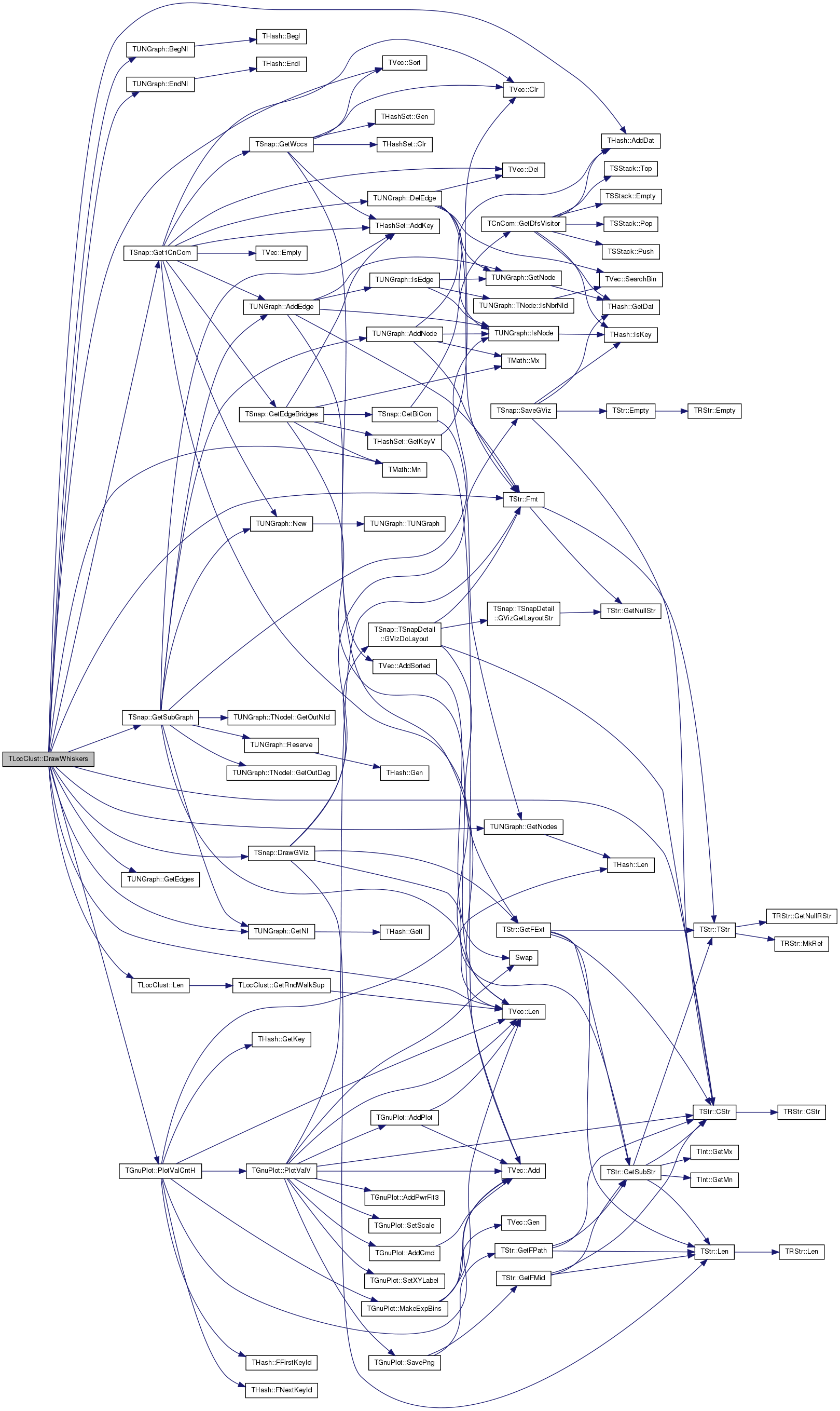void TLocClust::FindBestCut ( const int & SeedNode, const int & ClustSz, const double & MinSizeFrac = `0.2` )

Finds minimum conductance cut in the graph around the seed node.

Function first computes the ApproxPageRank(), initializes the SupportSweep() and then find the minimum conductance cluster. Parameter ClustSz controls the expected cluster size and is used to determine the tolerance (Eps) of the approximate PageRank calculation.

Definition at line 81 of file ncp.cpp.

Referenced by TLocClustStat::Run().

81  {
82  double MaxPhi = TFlt::Mx;
83  BestCutIdx = -1;
84  SeedNId = SeedNode;
85  // calculate pagerank and cut sets
86  ApproxPageRank(SeedNId, 1.0/double(ClustSz));
87  for (int i = 0; i < ProbH.Len(); i++) {
88  ProbH[i] /= Graph->GetNI(ProbH.GetKey(i)).GetOutDeg(); }
89  ProbH.SortByDat(false);
90  SupportSweep();
91  // find best cut
92  NIdV.Clr(false);
93  for (int i = 0; i < PhiV.Len(); i++) {
94  const double Phi = PhiV[i];
96  if (Phi < MaxPhi) { MaxPhi = Phi; BestCutIdx = i; }
97  }
98 }
int BestCutIdx
Definition: ncp.h:37
void SupportSweep()
After the function ApproxPageRank() has been run the SupportSweep() computes the volume, cut size, node ids, conductance vectors.
Definition: ncp.cpp:52
TFltV PhiV
Definition: ncp.h:36
int ApproxPageRank(const int &SeedNode, const double &Eps)
Computes Approximate PageRank from the seed node SeedNId and with tolerance Eps.
Definition: ncp.cpp:9
TSizeTy Len() const
Returns the number of elements in the vector.
Definition: ds.h:575
static const double Mx
Definition: dt.h:1391
void Clr(const bool &DoDel=true, const TSizeTy &NoDelLim=-1)
Clears the contents of the vector.
Definition: ds.h:1022
TNodeI GetNI(const int &NId) const
Returns an iterator referring to the node of ID NId in the graph.
Definition: graph.h:241
TIntV NIdV
Definition: ncp.h:35
PUNGraph Graph
Definition: ncp.h:28
int SeedNId
Definition: ncp.h:33
Adds a new element at the end of the vector, after its current last element.
Definition: ds.h:602
int Len() const
Definition: hash.h:228
const TKey & GetKey(const int &KeyId) const
Definition: hash.h:252
TIntFltH ProbH
Definition: ncp.h:30
void SortByDat(const bool &Asc=true)
Definition: hash.h:292

Here is the call graph for this function: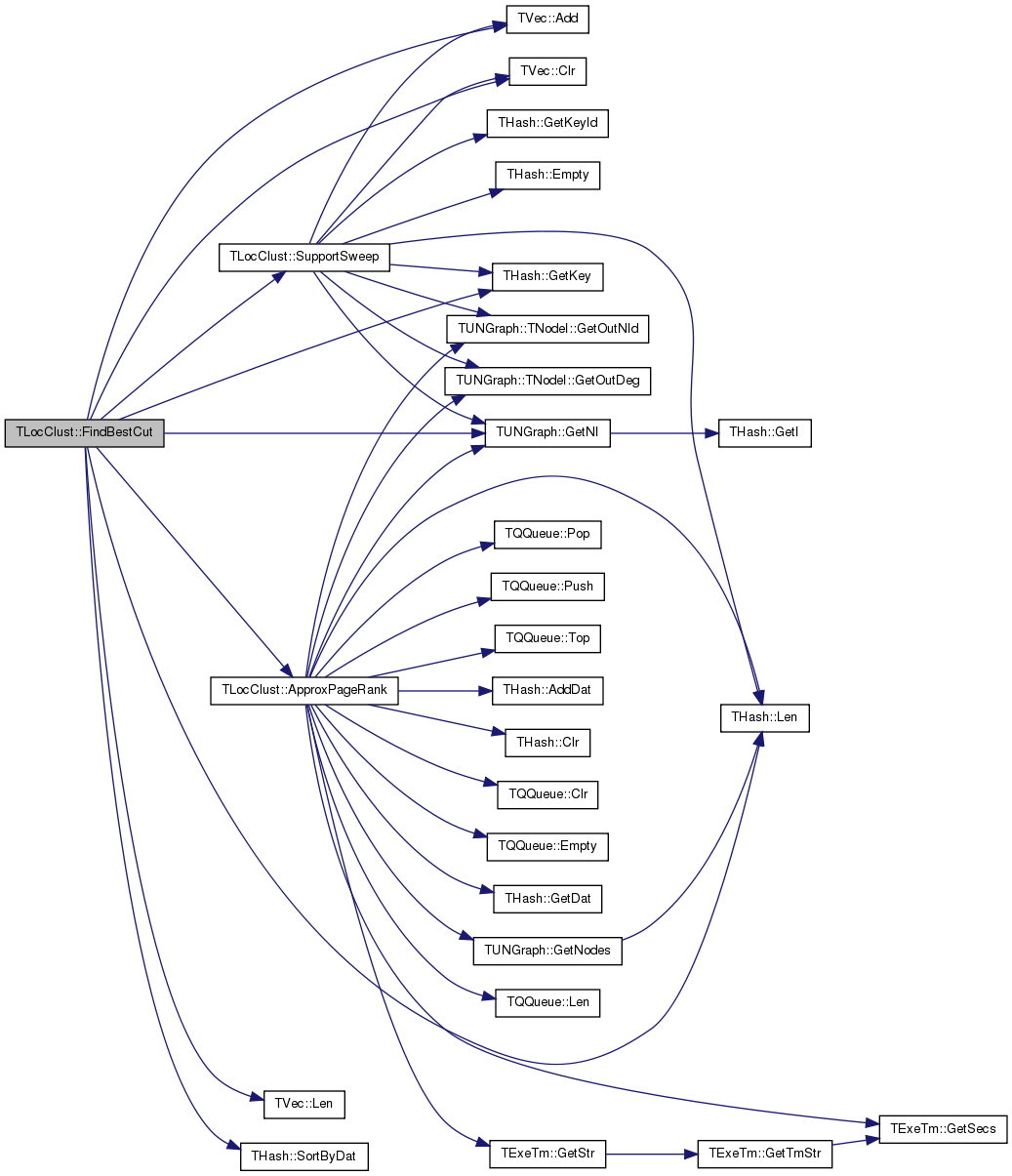Here is the caller graph for this function:int TLocClust::GetCut ( const int & Nodes ) const
inline

Returns the size of the cut of the first Nodes nodes in the sweep vector.

Size of the cut is the number of edges pointing between the first Nodes nodes and the remainder of the graph.

Definition at line 55 of file ncp.h.

References Nodes.

Referenced by GetCutEdges(), and TLocClustStat::Run().

55 { return CutV[Nodes]; }
int Nodes
Definition: ncp.h:29
TIntV CutV
Definition: ncp.h:35

Here is the caller graph for this function:int TLocClust::GetCutEdges ( ) const
inline

Number of edges in the 'best' (minimum conductance) cut.

Definition at line 77 of file ncp.h.

References BestCut(), and GetCut().

77 { return GetCut(BestCut()); }
int GetCut(const int &Nodes) const
Returns the size of the cut of the first Nodes nodes in the sweep vector.
Definition: ncp.h:55
int BestCut() const
Index K of the cut of the minimum conductance around the seed node.
Definition: ncp.h:73

Here is the call graph for this function:double TLocClust::GetCutPhi ( ) const
inline

Conductance of the 'best' (minimum conductance) cut.

Definition at line 81 of file ncp.h.

References BestCut(), and GetPhi().

Referenced by TLocClustStat::Run().

81 { return GetPhi(BestCut()); }
double GetPhi(const int &ValId) const
Returns the conductance of the cut separating the first Nodes nodes in the sweep vector from the rest...
Definition: ncp.h:59
int BestCut() const
Index K of the cut of the minimum conductance around the seed node.
Definition: ncp.h:73

Here is the call graph for this function:Here is the caller graph for this function:void TLocClust::GetCutStat ( const PUNGraph & Graph, const TIntV & NIdV, int & Vol, int & Cut, double & Phi, int GraphEdges = `-1` )
static

For a given Graph and a set of nodes NIdV the function returns the Volume, CutSize and the Conductance of the cut.

Definition at line 192 of file ncp.cpp.

192  {
193  TIntSet NIdSet(NIdV.Len());
194  for (int i = 0; i < NIdV.Len(); i++) { NIdSet.AddKey(NIdV[i]); }
195  GetCutStat(Graph, NIdSet, Vol, Cut, Phi, GraphEdges);
196 }
static void GetCutStat(const PUNGraph &Graph, const TIntV &NIdV, int &Vol, int &Cut, double &Phi, int GraphEdges=-1)
For a given Graph and a set of nodes NIdV the function returns the Volume, CutSize and the Conductanc...
Definition: ncp.cpp:192
TSizeTy Len() const
Returns the number of elements in the vector.
Definition: ds.h:575
Definition: shash.h:1254

Here is the call graph for this function:Here is the caller graph for this function: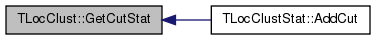void TLocClust::GetCutStat ( const PUNGraph & Graph, const TIntSet & NIdSet, int & Vol, int & Cut, double & Phi, int GraphEdges = `-1` )
static

For a given Graph and a set of nodes NIdV the function returns the Volume, CutSize and the Conductance of the cut.

Definition at line 198 of file ncp.cpp.

198  {
199  const int Edges2 = GraphEdges>=0 ? 2*GraphEdges : Graph->GetEdges();
200  Vol=0; Cut=0; Phi=0.0;
201  for (int i = 0; i < NIdSet.Len(); i++) {
202  if (! Graph->IsNode(NIdSet[i])) { continue; }
203  TUNGraph::TNodeI NI = Graph->GetNI(NIdSet[i]);
204  for (int e = 0; e < NI.GetOutDeg(); e++) {
205  if (! NIdSet.IsKey(NI.GetOutNId(e))) { Cut += 1; }
206  }
207  Vol += NI.GetOutDeg();
208  }
209  // get conductance
210  if (Vol != Edges2) {
211  if (2*Vol > Edges2) { Phi = Cut / double(Edges2-Vol); }
212  else if (Vol == 0) { Phi = 0.0; }
213  else { Phi = Cut / double(Vol); }
214  } else {
215  if (Vol == Edges2) { Phi = 1.0; }
216  }
217 }
Node iterator. Only forward iteration (operator++) is supported.
Definition: graph.h:68
bool IsKey(const TKey &Key) const
Definition: shash.h:1148
int GetOutDeg() const
Returns out-degree of the current node (returns same as value GetDeg() since the graph is undirected)...
Definition: graph.h:94
int Edges2
Definition: ncp.h:29
int Len() const
Definition: shash.h:1121
int GetOutNId(const int &NodeN) const
Returns ID of NodeN-th out-node (the node the current node points to).
Definition: graph.h:106

Here is the call graph for this function: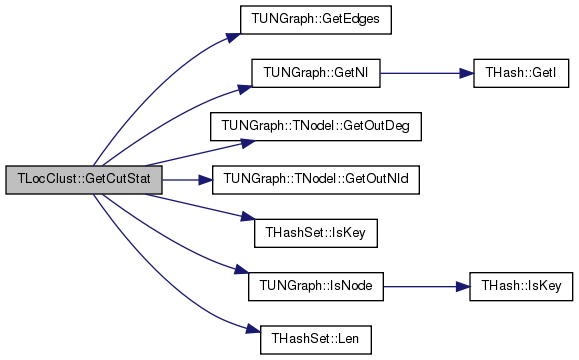const TIntV& TLocClust::GetCutV ( ) const
inline

Edges cut to separate the first K-nodes in the node-id vector (GetNIdV()) from the rest of the graph.

Definition at line 66 of file ncp.h.

References CutV.

66 { return CutV; }
TIntV CutV
Definition: ncp.h:35
 int TLocClust::GetCutVol ( ) const
inline

Volume of the 'best' (minimum conductance) cut.

Definition at line 79 of file ncp.h.

References BestCut(), and GetVol().

Referenced by TLocClustStat::Run().

79 { return GetVol(BestCut()); }
int GetVol(const int &Nodes) const
Returns the volume of the set of first NodeN nodes in the sweep vector.
Definition: ncp.h:51
int BestCut() const
Index K of the cut of the minimum conductance around the seed node.
Definition: ncp.h:73

Here is the call graph for this function:Here is the caller graph for this function:int TLocClust::GetNId ( const int & NodeN ) const
inline

Returns the ID of the NodeN-th node in the sweep vector.

Definition at line 47 of file ncp.h.

47 { return NIdV[NodeN]; }
TIntV NIdV
Definition: ncp.h:35
 const TIntV& TLocClust::GetNIdV ( ) const
inline

Vector of node IDs sorted in the random walk order.

Definition at line 62 of file ncp.h.

References NIdV.

Referenced by TLocClustStat::Run().

62 { return NIdV; }
TIntV NIdV
Definition: ncp.h:35

Here is the caller graph for this function:double TLocClust::GetPhi ( const int & ValId ) const
inline

Returns the conductance of the cut separating the first Nodes nodes in the sweep vector from the rest of the graph.

Conductance is the ration Cut/Volume. The lower the conductance the 'better' the cluster (higher volume, less edges cut).

Definition at line 59 of file ncp.h.

Referenced by GetCutPhi(), and TLocClustStat::Run().

59 { return PhiV[ValId]; }
TFltV PhiV
Definition: ncp.h:36

Here is the caller graph for this function:const TFltV& TLocClust::GetPhiV ( ) const
inline

Conducatance of the cut separating first K-nodes in the node-id vector (GetNIdV()) from the rest of the graph.

Definition at line 68 of file ncp.h.

References PhiV.

Referenced by TLocClustStat::Run().

68 { return PhiV; }
TFltV PhiV
Definition: ncp.h:36

Here is the caller graph for this function:int TLocClust::GetRndWalkSup ( ) const
inline

Returns the support of the approximate random walk, the number of nodes with non-zero PageRank score.

Definition at line 44 of file ncp.h.

References TVec< TVal, TSizeTy >::Len().

Referenced by Len().

44 { return VolV.Len(); }
TSizeTy Len() const
Returns the number of elements in the vector.
Definition: ds.h:575
TIntV VolV
Definition: ncp.h:35

Here is the call graph for this function: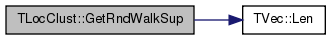Here is the caller graph for this function:int TLocClust::GetVol ( const int & Nodes ) const
inline

Returns the volume of the set of first NodeN nodes in the sweep vector.

Volume is defined as the sum of the degrees of the first Nodes nodes. Or in other words volume = 2* edges inside the set + the edges pointing outside the set.

Definition at line 51 of file ncp.h.

References Nodes.

Referenced by GetCutVol(), and TLocClustStat::Run().

51 { return VolV[Nodes]; }
TIntV VolV
Definition: ncp.h:35
int Nodes
Definition: ncp.h:29

Here is the caller graph for this function:const TIntV& TLocClust::GetVolV ( ) const
inline

Volume (sum of the degrees) of first K-nodes in the node-id vector (GetNIdV()).

Definition at line 64 of file ncp.h.

References VolV.

64 { return VolV; }
TIntV VolV
Definition: ncp.h:35
 int TLocClust::Len ( ) const
inline

Returns the support of the approximate random walk, the number of nodes with non-zero PageRank score.

Definition at line 42 of file ncp.h.

References GetRndWalkSup().

Referenced by DrawWhiskers(), and TLocClustStat::Run().

42 { return GetRndWalkSup(); }
int GetRndWalkSup() const
Returns the support of the approximate random walk, the number of nodes with non-zero PageRank score...
Definition: ncp.h:44

Here is the call graph for this function: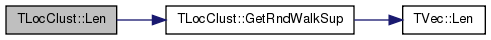Here is the caller graph for this function: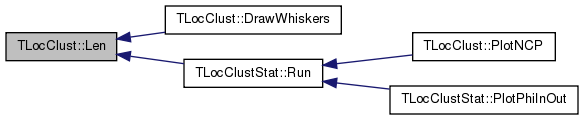void TLocClust::PlotCutDistr ( const TStr & OutFNm, TStr Desc = `TStr()` ) const

Plots the cluster cut size vs. cluster size K (cluster is composed of nodes NIdV[1...K]).

Definition at line 113 of file ncp.cpp.

113  {
114  TFltPrV RankValV(CutV.Len(), 0);
115  for (int i = 0; i < CutV.Len(); i++) {
117  if (Desc.Empty()) { Desc = OutFNm; }
118  TFltPrV NewV; TLocClustStat::MakeExpBins(RankValV, NewV);
119  TGnuPlot GP(OutFNm+"-CUT", TStr::Fmt("CUT SIZE. Seed node %d.", SeedNId, Desc.CStr()));
120  GP.AddPlot(NewV, gpwLinesPoints, ""); //, "pointtype 6 pointsize 1.5"
121  GP.SetXYLabel("Rank", "Cut size");
122  //GP.SetScale(gpsLog10X);
123  GP.SavePng();
124 }
TSizeTy Len() const
Returns the number of elements in the vector.
Definition: ds.h:575
static void MakeExpBins(const TFltPrV &ValV, TFltPrV &NewV)
Definition: ncp.cpp:1020
TPair< TFlt, TFlt > TFltPr
Definition: ds.h:99
TIntV CutV
Definition: ncp.h:35
bool Empty() const
Definition: dt.h:491
static TStr Fmt(const char *FmtStr,...)
Definition: dt.cpp:1599
int SeedNId
Definition: ncp.h:33
char * CStr()
Definition: dt.h:479
Adds a new element at the end of the vector, after its current last element.
Definition: ds.h:602
Vector is a sequence TVal objects representing an array that can change in size.
Definition: ds.h:430

Here is the call graph for this function: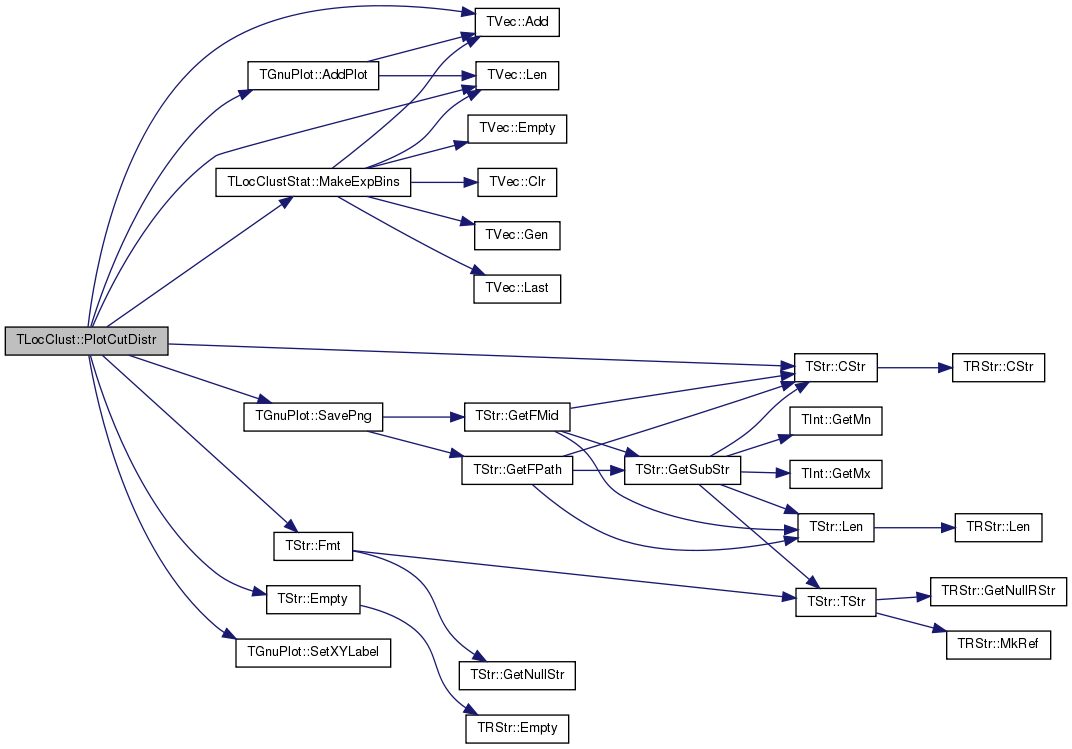void TLocClust::PlotNCP ( const PUNGraph & Graph, const TStr & FNm, const TStr Desc = `""`, const bool & BagOfWhiskers = `true`, const bool & RewireNet = `false`, const int & KMin = `10`, const int & KMax = `Mega(100)`, const int & Coverage = `10`, const bool & SaveTxtStat = `false`, const bool & PlotBoltzman = `false` )
static

Plots the Network Community Profile (NCP) of a given graph Graph. The NCP plot of a network captures the global community structure of the network. The NCP plot of a network captures the global community structure of the network. Refer to 'Community Structure in Large Networks: Natural Cluster Sizes and the Absence of Large Well-Defined Clusters by J. Leskovec, K. Lang, A. Dasgupta, M. Mahoney. Internet Mathematics 6(1) 29–123, 2009' for the explanation of how to read these plots. URL: http://arxiv.org/abs/0810.1355

Definition at line 219 of file ncp.cpp.

219  {
220  const double Alpha = 0.001, KFac = 1.5, SizeFrac = 0.001;
221  //const int KMin = 2, KMax = Mega(100), Coverage = 10;
222  TLocClustStat ClusStat1(Alpha, KMin, KMax, KFac, Coverage, SizeFrac);
223  ClusStat1.Run(Graph, false, PlotBoltzman, SaveTxtStat);
224  if (BagOfWhiskers) { ClusStat1.AddBagOfWhiskers(); }
225  TLocClustStat ClusStat2(Alpha, KMin, KMax, KFac, Coverage, SizeFrac);
226  ClusStat1.ImposeNCP(ClusStat2, FNm, Desc, "ORIGINAL", "REWIRED"); // plot before rewiring
227  if (SaveTxtStat) { // for every piece size store modularity
228  ClusStat1.SaveTxtInfo(FNm, Desc, false);
229  }
230  if (PlotBoltzman) {
231  ClusStat1.PlotBoltzmanCurve(FNm, Desc);
232  }
233  if (RewireNet) {
234  ClusStat2.Run(TSnap::GenRewire(Graph, 50, TInt::Rnd), false, false, false);
235  if (BagOfWhiskers) { ClusStat2.AddBagOfWhiskers(); }
236  ClusStat1.ImposeNCP(ClusStat2, FNm, Desc, "ORIGINAL", "REWIRED"); // if rewire, plot again
237  }
238 }
PUNGraph GenRewire(const PUNGraph &OrigGraph, const int &NSwitch, TRnd &Rnd)
Rewire a random undirected graph. Keeps node degrees the same, but randomly rewires the edges...
Definition: ggen.cpp:168
static TRnd Rnd
Definition: dt.h:1146
Local-Spectral-Clustering statistics of a given Graph.
Definition: ncp.h:125
double Alpha
Definition: ncp.h:32

Here is the call graph for this function: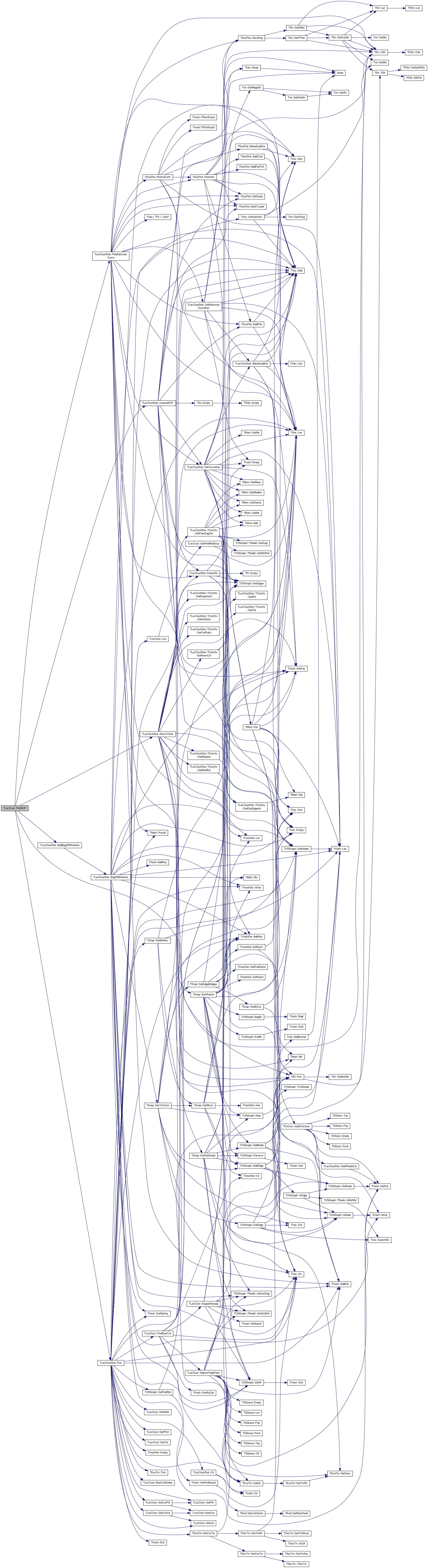void TLocClust::PlotPhiDistr ( const TStr & OutFNm, TStr Desc = `TStr()` ) const

Plots the cluster conductance vs. cluster size K (cluster is composed of nodes NIdV[1...K]).

Definition at line 126 of file ncp.cpp.

126  {
127  TFltPrV RankValV(PhiV.Len(), 0);
128  for (int i = 0; i < PhiV.Len(); i++) {
130  if (Desc.Empty()) { Desc = OutFNm; }
131  TFltPrV NewV; TLocClustStat::MakeExpBins(RankValV, NewV);
132  TGnuPlot GP(OutFNm+"-PHI", TStr::Fmt("CONDUCTANCE (Cut size / Volume). Seed node %d.", SeedNId, Desc.CStr()));
133  GP.AddPlot(NewV, gpwLinesPoints, ""); //, "pointtype 6 pointsize 1.5"
134  GP.SetXYLabel("Rank", "Conductance (Cut size / Volume)");
135  //GP.SetScale(gpsLog10X);
136  GP.SavePng();
137 }
TFltV PhiV
Definition: ncp.h:36
TSizeTy Len() const
Returns the number of elements in the vector.
Definition: ds.h:575
static void MakeExpBins(const TFltPrV &ValV, TFltPrV &NewV)
Definition: ncp.cpp:1020
TPair< TFlt, TFlt > TFltPr
Definition: ds.h:99
bool Empty() const
Definition: dt.h:491
static TStr Fmt(const char *FmtStr,...)
Definition: dt.cpp:1599
int SeedNId
Definition: ncp.h:33
char * CStr()
Definition: dt.h:479
Adds a new element at the end of the vector, after its current last element.
Definition: ds.h:602
Vector is a sequence TVal objects representing an array that can change in size.
Definition: ds.h:430

Here is the call graph for this function: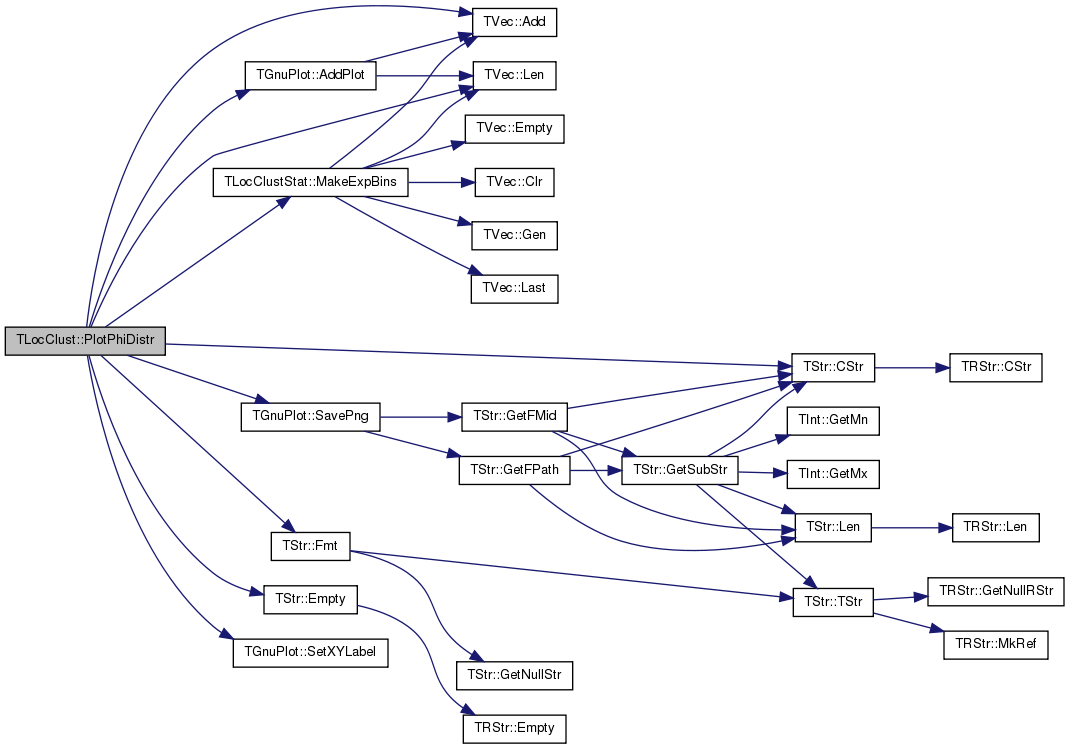void TLocClust::PlotVolDistr ( const TStr & OutFNm, TStr Desc = `TStr()` ) const

Plots the cluster volume vs. cluster size K (cluster is composed of nodes NIdV[1...K]).

Definition at line 100 of file ncp.cpp.

100  {
101  TFltPrV RankValV(VolV.Len(), 0);
102  for (int i = 0; i < VolV.Len(); i++) {
104  if (Desc.Empty()) { Desc = OutFNm; }
105  TFltPrV NewV; TLocClustStat::MakeExpBins(RankValV, NewV);
106  TGnuPlot GP(OutFNm+"-VOL", TStr::Fmt("VOLUME. Seed node %d.", SeedNId, Desc.CStr()));
107  GP.AddPlot(NewV, gpwLinesPoints, ""); //, "pointtype 6 pointsize 1.5"
108  GP.SetXYLabel("Rank", "Volume");
109  //GP.SetScale(gpsLog10X);
110  GP.SavePng();
111 }
TSizeTy Len() const
Returns the number of elements in the vector.
Definition: ds.h:575
TIntV VolV
Definition: ncp.h:35
static void MakeExpBins(const TFltPrV &ValV, TFltPrV &NewV)
Definition: ncp.cpp:1020
TPair< TFlt, TFlt > TFltPr
Definition: ds.h:99
bool Empty() const
Definition: dt.h:491
static TStr Fmt(const char *FmtStr,...)
Definition: dt.cpp:1599
int SeedNId
Definition: ncp.h:33
char * CStr()
Definition: dt.h:479
Adds a new element at the end of the vector, after its current last element.
Definition: ds.h:602
Vector is a sequence TVal objects representing an array that can change in size.
Definition: ds.h:430

Here is the call graph for this function: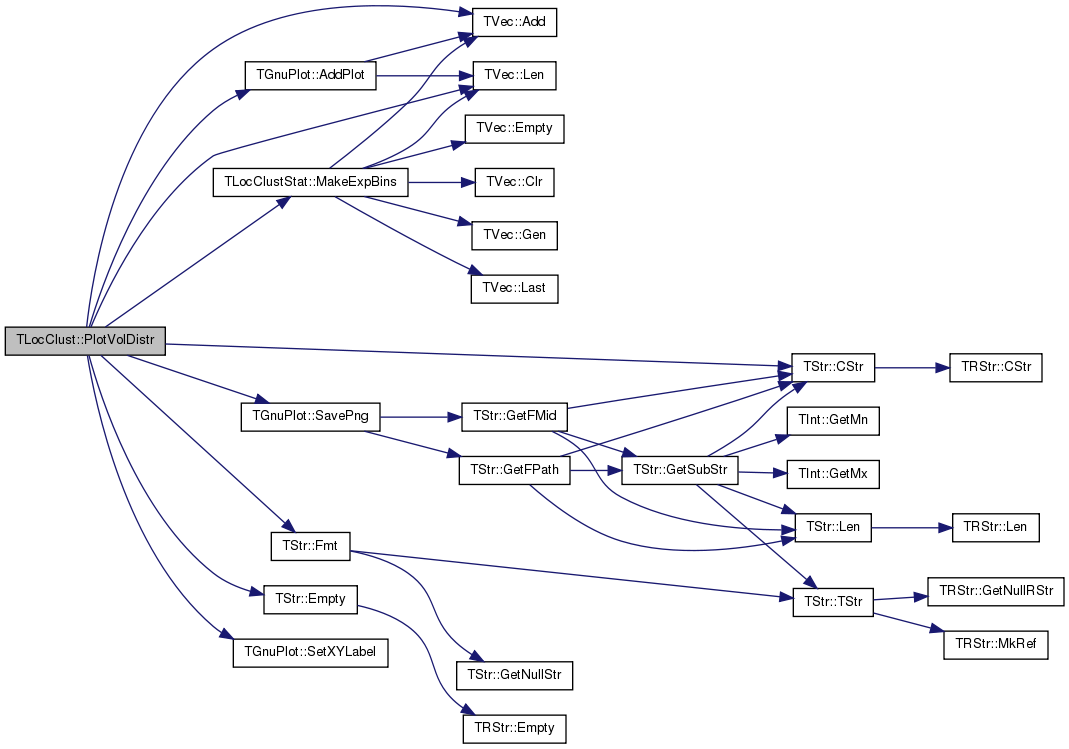void TLocClust::SavePajek ( const TStr & OutFNm ) const

Saves the network in the Pajek format so it can be visualized. Red node represents the seed and color the cluster membership.

Definition at line 139 of file ncp.cpp.

139  {
140  FILE *F = fopen(TStr::Fmt("%s.net", OutFNm.CStr()).CStr(), "wt");
141  TIntH NIdToIdH(Graph->GetNodes(), true);
142  TIntH ClustSet(BestCut()+1);
143  const int BucketSz = BestCutNodes()/ 4;
144  TStrV ClrV = TStrV::GetV("Black", "Gray80", "Gray60", "Gray40", "Gray20", "RedViolet");
145  for (int a = 0; a < BestCutNodes(); a++) {
147  }
148  fprintf(F, "*Vertices %d\n", Graph->GetNodes());
149  int i = 0;
150  for (TUNGraph::TNodeI NI = Graph->BegNI(); NI < Graph->EndNI(); NI++) {
151  const int NId = NI.GetId();
152  if (NId == SeedNId) {
153  fprintf(F, "%d \"%d\" diamond x_fact 2 y_fact 2 ic Green fos 10\n", i+1, NId); }
154  else if (ClustSet.IsKey(NId)) {
155  //fprintf(F, "%d \"%d\" box x_fact 1 y_fact 1 ic Red fos 10\n", i+1, NId); }
156  fprintf(F, "%d \"%d\" box x_fact 2 y_fact 2 ic %s fos 10\n", i+1, NId, ClrV[ClustSet.GetDat(NId)].CStr()); }
157  else {
158  fprintf(F, "%d \"%d\" ellipse x_fact 2 y_fact 2 ic Blue fos 10\n", i+1, NId); }
160  i++;
161  }
162  fprintf(F, "*Arcs %d\n", Graph->GetEdges()); // arcs are directed, edges are undirected
163  for (TUNGraph::TNodeI NI = Graph->BegNI(); NI < Graph->EndNI(); NI++) {
164  const int NId = NIdToIdH.GetDat(NI.GetId());
165  for (int e = 0; e < NI.GetOutDeg(); e++) {
166  fprintf(F, "%d %d %g c Tan\n", NId, NIdToIdH.GetDat(NI.GetOutNId(e)).Val, 1.0);
167  }
168  }
169  fclose(F);
170 }
int GetEdges() const
Returns the number of edges in the graph.
Definition: graph.cpp:82
Node iterator. Only forward iteration (operator++) is supported.
Definition: graph.h:68
int GetNodes() const
Returns the number of nodes in the graph.
Definition: graph.h:192
TIntV NIdV
Definition: ncp.h:35
static TStr Fmt(const char *FmtStr,...)
Definition: dt.cpp:1599
PUNGraph Graph
Definition: ncp.h:28
TNodeI EndNI() const
Returns an iterator referring to the past-the-end node in the graph.
Definition: graph.h:239
int BestCut() const
Index K of the cut of the minimum conductance around the seed node.
Definition: ncp.h:73
int SeedNId
Definition: ncp.h:33
static TVec< TStr, int > GetV(const TStr &Val1)
Returns a vector on element Val1.
Definition: ds.h:848
char * CStr()
Definition: dt.h:479
TNodeI BegNI() const
Returns an iterator referring to the first node in the graph.
Definition: graph.h:237
int BestCutNodes() const
Number of nodes inside the 'best' (minimum conductance) cut.
Definition: ncp.h:75

Here is the call graph for this function: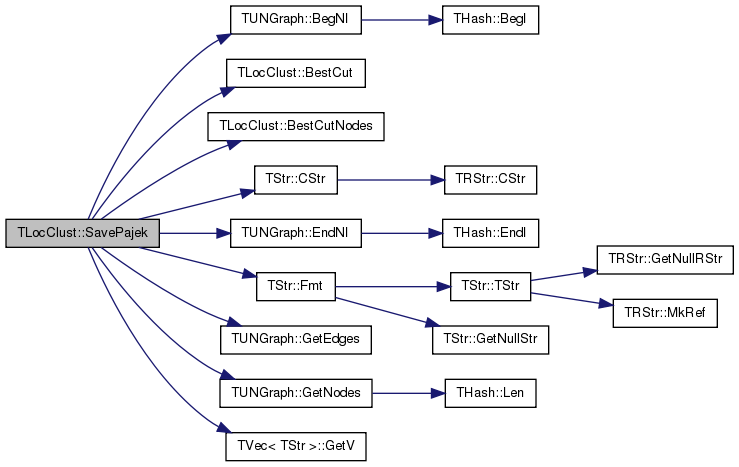void TLocClust::SupportSweep ( )

After the function ApproxPageRank() has been run the SupportSweep() computes the volume, cut size, node ids, conductance vectors.

Definition at line 52 of file ncp.cpp.

Referenced by FindBestCut().

52  {
53  TExeTm ExeTm;
54  VolV.Clr(false); CutV.Clr(false); PhiV.Clr(false);
55  if (ProbH.Empty()) { return; }
56  const int TopNId = ProbH.GetKey(0);
57  const int TopNIdDeg = Graph->GetNI(TopNId).GetOutDeg();
58  int Vol = TopNIdDeg, Cut = TopNIdDeg;
59  double Phi = Cut/double(Vol);
61  for (int i = 1; i < ProbH.Len(); i++) {
62  const int NId = ProbH.GetKey(i);
63  const TUNGraph::TNodeI& Node = Graph->GetNI(NId);
64  const int OutDeg = Node.GetOutDeg();
65  int CutSz = OutDeg; // edges outside
66  for (int e = 0; e < OutDeg; e++) {
67  const int Rank = ProbH.GetKeyId(Node.GetOutNId(e));
68  if ( Rank > -1 && Rank < i) { CutSz -= 2; }
69  }
70  Vol += OutDeg; Cut += CutSz;
71  if (Vol < Edges2) {
72  if (2*Vol > Edges2) { Phi = Cut / double(Edges2-Vol); }
73  else { Phi = Cut / double(Vol); }
74  } else {
75  Phi = 1.0;
76  }
77  IAssert((Phi+1e-6) >= double(1.0)/double(i*(i+1)+1)); // conductance is worse than the best possible
79  }}
#define IAssert(Cond)
Definition: bd.h:262
Definition: tm.h:355
TFltV PhiV
Definition: ncp.h:36
Node iterator. Only forward iteration (operator++) is supported.
Definition: graph.h:68
bool Empty() const
Definition: hash.h:227
int GetOutDeg() const
Returns out-degree of the current node (returns same as value GetDeg() since the graph is undirected)...
Definition: graph.h:94
void Clr(const bool &DoDel=true, const TSizeTy &NoDelLim=-1)
Clears the contents of the vector.
Definition: ds.h:1022
int Edges2
Definition: ncp.h:29
TNodeI GetNI(const int &NId) const
Returns an iterator referring to the node of ID NId in the graph.
Definition: graph.h:241
TIntV VolV
Definition: ncp.h:35
int GetKeyId(const TKey &Key) const
Definition: hash.h:466
TIntV CutV
Definition: ncp.h:35
int GetOutNId(const int &NodeN) const
Returns ID of NodeN-th out-node (the node the current node points to).
Definition: graph.h:106
PUNGraph Graph
Definition: ncp.h:28
Adds a new element at the end of the vector, after its current last element.
Definition: ds.h:602
int Len() const
Definition: hash.h:228
const TKey & GetKey(const int &KeyId) const
Definition: hash.h:252
TIntFltH ProbH
Definition: ncp.h:30

Here is the call graph for this function: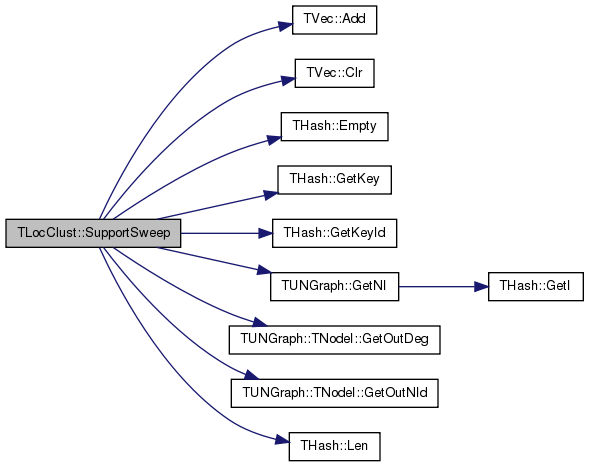Here is the caller graph for this function:## Friends And Related Function Documentation

 friend class TLocClustStat
friend

Definition at line 120 of file ncp.h.

## Member Data Documentation

 double TLocClust::Alpha
private

Definition at line 32 of file ncp.h.

Referenced by ApproxPageRank(), and PlotNCP().

 int TLocClust::BestCutIdx
private

Definition at line 37 of file ncp.h.

Referenced by BestCut(), and FindBestCut().

 TIntV TLocClust::CutV
private

Definition at line 35 of file ncp.h.

Referenced by GetCutV(), PlotCutDistr(), and SupportSweep().

 int TLocClust::Edges2
private

Definition at line 29 of file ncp.h.

Referenced by GetCutStat(), and SupportSweep().

 PUNGraph TLocClust::Graph
private

Definition at line 28 of file ncp.h.

Referenced by ApproxPageRank(), FindBestCut(), SavePajek(), and SupportSweep().

 TIntV TLocClust::NIdV
private

Definition at line 35 of file ncp.h.

Referenced by DrawWhiskers(), FindBestCut(), GetNIdV(), and SavePajek().

 TIntQ TLocClust::NodeQ
private

Definition at line 31 of file ncp.h.

Referenced by ApproxPageRank().

 int TLocClust::Nodes
private

Definition at line 29 of file ncp.h.

Referenced by GetCut(), and GetVol().

 TFltV TLocClust::PhiV
private

Definition at line 36 of file ncp.h.

Referenced by FindBestCut(), GetPhiV(), PlotPhiDistr(), and SupportSweep().

 TIntFltH TLocClust::ProbH
private

Definition at line 30 of file ncp.h.

Referenced by ApproxPageRank(), FindBestCut(), and SupportSweep().

 TIntFltH TLocClust::ResH
private

Definition at line 30 of file ncp.h.

Referenced by ApproxPageRank().

 int TLocClust::SeedNId
private

Definition at line 33 of file ncp.h.

Referenced by FindBestCut(), PlotCutDistr(), PlotPhiDistr(), PlotVolDistr(), and SavePajek().

 bool TLocClust::Verbose = true
static

Definition at line 26 of file ncp.h.

Referenced by TLocClustStat::Run().

 TIntV TLocClust::VolV
private

Definition at line 35 of file ncp.h.

Referenced by GetVolV(), PlotVolDistr(), and SupportSweep().

The documentation for this class was generated from the following files: